Main Page

# Rubik's Magic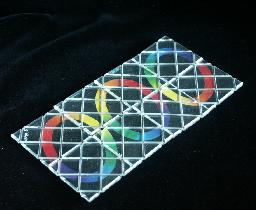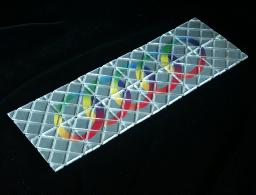This amazing puzzle consists of a number of tiles arranged in a rectangle. They are connected to each other by wires that run in diagonal grooves on the tiles alternatively under and over them. If two of the tiles lie on top of each other, the wires allow them to be unfolded in two perpendicular directions provided connections to the other tiles do not restrict the movements. The kind of wire arrangement can also be found in certain wallets which can be opened in two different directions because of the ribbons connecting the two halves.

In the rectangular starting position the front of the tiles show a complete picture. The other side however shows a mixed up picture. The aim is of course to rearrange the tiles to unscramble this second picture. In most versions of Rubik's Magic the solved shape is not rectangular.

Ernő Rubik was granted a US patent on 11 August 1987 (US 4,685,680), but there is an earlier Hungarian patent (19 Mar 1985, HU 1211/85).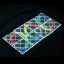The original version of Rubik's Magic is a black 2×4 rectangle with rainbow coloured rings, and was made by Matchbox in 1986. It has recently been released again, this time manufactured by Oddzon, but now it is red with yellow rings.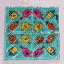In 1987 Matchbox made several other versions. There were for example Mini Magics that are 2×2 squares of tiles. These are quite rare.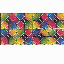Another fairly rare 1987 version of Rubik's Magic was 'Create the Cube', which had 8 differently coloured tiles, and the aim was to make the cube shape so that the colours matched.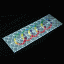There was also the Master Edition which has 2×6 silver coloured tiles and has 5 rings on each side and, unlike the the normal magic, the rings must be unlinked.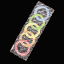Unofficial versions of the Magic were made too, such as the Magic Rings which is a 2×6 magic and its solved position is on the shape of a cross.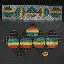A Polish version called the Magic Balls is 2×8 and has smaller tiles than the official Magics.

## How the tiles hinge.

Each tile is connected to exactly two others. For the moment lets just consider two connected tiles in isolation. Fold one on top of the other. There will now always be two ways the top one can be unfolded; either back the same way, or in a direction perpendicular to that. Which way this second folding direction goes depends on the wires, but it will always be at 90 degrees with the first folding direction. Of course the folding may not be possible due to the constraints imposed by the other tiles of the puzzle. Suppose that you have two tiles on top of one another, and that the top tile could folds downwards or rightwards. If you manage to fold the top tile downwards and swing it underneath the other tile, then it will now fold leftwards instead. This is always true; if a tile is folded around, its other folding direction changes to the opposite direction.

## The number of positions:

There are obviously an infinite number of three dimensional shapes, but the number of flat patterns is limited. First you must realise that the tiles always remain in the same order around the loop. If you number them 1-to-2n going clockwise around the rectangle in the starting position, then every other position will also have them in 1-to-2n order, starting from wherever tile 1 is, and going either clockwise or anti-clockwise around the rectangle. From the transforms it is clear that in a 2×n rectangle:

• Any tile can be in the top left corner. (2×n)
• The tiles must all be in matching orientation, but any orientation is possible. (4)
• The tiles can be in 1-to-2n or 2n-to-1 order around the loop. (2)

This leaves 2·n·4·2 = 16n possibilities. However the rectangle can be rotated 180 degrees, and those positions have been counted as different. Therefore there are only 8×n different possible rectangles.
The same argument applies to any flat shape, so any non-symmetric flat shape has 16n possible patterns, and flat shapes with rotational symmetry only 8n (or even 4n if it has 90 degree rotational symmetry). In a later section on this page we will enumerate which flat shapes can actually be achieved on the normal and Master magics.

## Getting back to the starting position

Once you have a rectangle shape, the three main transforms can always get the puzzle back to its starting position. There is a fairly simply way to do this:

1. If the pieces all seem to need a half turn to line them up with the adjacent pieces in the loop, then do the rectangle transform.
2. If the pieces all seem to need a quarter turn, then:
1. Look at the two tiles at one end of the rectangle.
2. If their edges don't match, do the loop shift transform shifting along one tile.
3. Do the rectangle transform.
3. If the top and bottom rows are upside down, then do the loop shift transform shifting along one tile.
4. Use the star shift transform to shift the top/bottom pieces to their own halves.

Here is a neat diagram showing how the rectangle configurations are related to each other by the three transforms.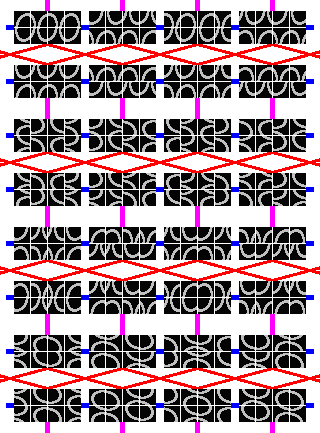In the procedure above, step a makes sure that arrangement is not one of those in rows 5 or 6 in the diagram, step b2 eliminates rows 4 and 7, and then b3 rows 3 and 6. Steps c and d reach the solved state from anywhere in rows 1 and 2.

## Flat shapes

It is interesting to find out which flat shapes can be made with this puzzle. Obviously the pieces must be made from a loop of tiles, so each tile must be connected to exactly two other tiles. There is a further constraint, which for example prohibits the normal sized magic to form a 3×3 square with a hole in the middle.

Suppose you have the puzzle in a flat shape. Number the tiles of the loop with the numbers 1 to 2n. For all the odd numbered tiles, count the number of right turns the loop makes at those tiles, and subtract the number of left turns. Do the same for all the even numbered tiles. These two numbers must be equal for the pattern to be possible, and for it to be a flat closed loop they must both be two (or minus two if you numbered them anti-clockwise) because you need 4 quarter turns to go full circle.

It is fairly easy to see why this condition exists. It is true for the initial position of the puzzle. Every time two adjacent tiles move their connection from one edge to another, the condition still holds. Therefore it must be true for every possible shape. (Note that for this reasoning to offer a valid proof, the condition should be extended and formalised so that it does not depend on the puzzle being a flat shape and can handle positions with tiles that are folded onto each other, but is easily done.)

For example, consider the solved position of the Master Edition.

 12 1 11 2 3 10 9 4 5 8 7 6

With the odd numbered tiles there are right turns at 1, 3, 5 and a left turn at 9, so there are two more right turns than left.
With the even numbered tiles there are right turns at 6, 8, 10, 12 and left turns at 2, 4 so there are again two more right turns than left.

With this constraint, there are only 15 possible flat patterns left, and they can all be constructed. It makes a good exercise to try to construct them all.

 X X X X X X X X X X X X
 X X X X X X X X X X X X
 X X X X X X X X X X X X
 X X X X X X X X X X X X
 X X X X X X X X X X X X
 X X X X X X X X X X X X
 X X X X X X X X X X X X
 X X X X X X X X X X X X
 X X X X X X X X X X X X
 X X X X X X X X X X X X
 X X X X X X X X X X X X
 X X X X X X X X X X X X
 X X X X X X X X X X X X
 X X X X X X X X X X X X
 X X X X X X X X X X X X

An interesting consequence of the constraint above is that even with larger versions of the puzzle, its flat shapes can never enclose an odd area. In other words, if the flat shape has holes then their total area is the same as an even number of tiles. I think I have proved that the converse is also true, i.e. that if a flat shape made of a loop of 4n tiles has no holes, or has holes with an even total area, then the shape can be made from a rectangular magic of the right size.

## Making your own Master/Super Magic

It is possible to make the Magic Master Edition, or a 'Super Magic' yourself, by recombining two normal Rubik's Magics. The first time this is quite difficult to do, and not for the faint-hearted. After you have done it once or twice it becomes easier, but it will always be a lot of hard intricate work.

I made a Super Magic by connecting one old magic (black) and one new magic (red). If you look at a normal magic in the starting position (unlinked rings), three tiles at one end of the reverse side will be correct. By connecting the two Magics at those ends, it will be possible to solve the resulting Super Magic. On the front, the red magic is on the left, and the black magic on the right, with the copyright messages of both of them along the bottom. When solved, the reverse shows two sets of 3 linked rings. You can of course connect two Magics of the same type but then one has to be upside down, i.e. with the copyright message along the top edge (red Magics: copyright along top right and bottom left; black Magics: copyright along top left and bottom right). Of course, if you replace the design with one of your own as explained later, then none of the above is relevant.

The magic is made up of tiles which are held together by many loops of the same size. If the string loops are removed, the tiles fall apart in two halves, and the paper inside bearing the design can be removed. You can therefore replace the design with one of your own by replacing the paper inserts. I have made fairly accurate drawings of the designs for the Create the Cube, Magic Rings and the Magic Balls. You can download them here:
Create the Cube: back, front, or MSWord document (511Kb).
Magic Balls: back, front, solved, or MSWord document (79Kb).
Magic Rings: back left half, back right half, front left half, front right half (about 200Kb each)

Making your own design is difficult, unless you have a finished puzzle to try it out with. Then you can do the following steps to make your design:

1. Put the puzzle in the shape you want the solved position to be.
2. Make a design to match this shape for the solved position.
3. Mark the tiles, using small labels for example, so that you can later match the parts of the design with the tiles. Make sure you can recognize the orientation of the tiles as well.
4. Change the puzzle to a rectangle shape.
5. Make a design to match this rectangle shape for the starting position.
6. Use the markings on the tiles to match up the designs for the front and back.
7. Put together a new Magic puzzle with using these designs, as described below (or take apart and rebuild the puzzle you used).

To make a Rubik's magic, you need to acquire the tiles and string loops. There are two ways to get these. Either you can buy some Rubik's Magics and take them apart, or you can buy some DIY Magic kits from Rubik's site which consist of 8 tiles and 18 loops (16 plus 2 spare). I do not recommend using the tiles from a Magic Strategy Game (a two-player game using tiles that Rubik marketed at the same time as the Master Edition). These tiles are glued so it is hard to replace its design, there are no dents in the edges of the tiles to allow the loops to wrap around, and also you would still have to acquire the loops themselves.

The string loops are all of the same length, just long enough to wrap around three tiles. Once you know how to connect three tiles together with those loops, the same technique can be used to make a magic of any even number of tiles, so I will first describe how three tiles can be fully connected with 4 string loops.

• Each string should be in a groove, alternating between over and under a tile, and zigzagging between the edges of the rectangle like a reflecting beam of light.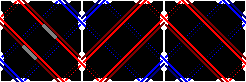• One pair of loops runs along one groove, the other pair along the other parallel groove.
• Before trying to put loops on, put together each of the three tiles, and use a bit of sellotape around the top and bottom edges to keep them whole. Leave the grooves free from the tape. Nowadays I don't bother with tape, but when doing the first few times it is a great help.
• Lay the first loop in the grooves of the left tile. Make sure however that the metal bit that connects the string ends to a loop lies in one of the long grooves of that left tile.
• Place one part of the string where it belongs in the groove of the middle tile, and around the right tile. Only a short part of the loop now remains, and it has to be pulled over one of the tile corners in order to be put in its proper place. Note that this last corner should not be one of the outer corners of the 1×3 rectangle, but one of the inner tile corners.
• Pulling the first loop around the last tile corner requires no force at all, since the tiles are still free to move about. You can twist one tile to give you enough slack in the loop to pull it over the corner.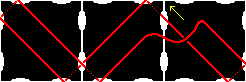• Put the second loop through the same groove as the first in exactly the same way, but keep in mind the following points:
1. Again make sure that the loop's metal bit is in a long groove of the left tile, but not the same one as the first loop's bit.
2. When you put the second loop on, make sure it twists in the opposite way between the tiles compared to the first string. In other words, if the strands of the first loop cross left over right, the strands of this second loop cross right over left. The crossing point between the tiles will then look like it has two strands going in between two others.• Turn the tiles upside down, so that the other side is now facing you. Put the third and fourth loops through the now visible empty grooves in exactly the same way as you did with the first two loops. The only difference is that these do require a littl force to snap in place. Again, always pull it over an inner corner, not one of the outer ones. Also, as you stretch the string, flex the tiles back and forth a little so that the tension is distributed over the whole loop, and not just the section you are pulling at. The metal bits should again be in separate long grooves of the left tile.

You can use this technique to build any size magic, as long as it has an even number of tiles. You will need twice as many loops as there are tiles. Note that Magics with a size that is not a multiple of 4 are also possible, and are quite different to play with.

1. Take all the separate tiles (each held together by some tape) and arrange them in the rectangular starting position.
2. Take the bottom row, and move it as a whole to the right, and rotate it through 180 degrees and place it to the right of the top row, making a long thin rectangle. On a normal magic, this would mean that the top halves of the unlinked rings are on the left, and the bottom halves are showing upside down on the right.
3. String tiles 1 to 3 of this rectangle (numbered from left to right) together as described above. Note that there are two ways of doing this, depending on the direction of the strings. Both of these lead to solvable puzzles, the only difference being that all the hinges will work the opposite way. On the old Master Edition and on the Magic Balls version of the Super Magic, the strings go towards the bottom left corner of tile 1 on the front side. On the front of the normal magic and on the new Master Edition however they go towards the top left corner.
4. String together tiles 3 to 5, in the same manner as before, using the same grooves on the sharecd tile 3 as the previous set of strings. Again, make sure all the metal bits go on the leftmost tile, i.e. tile 3.
5. String the rest of this rectangle together, by connecting tiles 5 to 7, 7 to 9 and so on. Continue until you have only one unconnected tile left.
6. Instead of connecting two loose tiles to the end, you now connect the last loose tile and tile 1, thus making one big loop.
7. The puzzle is now finished, so recover the starting position and start playing.

The above was the way the Magics used to be strung when they were first produced. Nowadays a slightly different method is used, that may be slightly easier. This technique goes as follows:

1. Take all the separate tiles (each held together by some tape) and arrange them in the rectangular starting position.
2. Take the bottom row, and move it as a whole to the right, and rotate it through 180 degrees and place it to the right of the top row, making a long thin rectangle. On a normal magic, this would mean that the top halves of the unlinked rings are on the left, and the bottom halves are showing upside down on the right.
3. String tiles 1 to 3 of this rectangle (numbered from left to right) together, but using ONLY ONE string loop on each groove. Put the metal bits in the lower of the two grooves on each side of tile 1.
4. String tiles 2 to 4 together, again with only one string loop in each groove. Note that this doubles the strings on tiles 2 and 3, and hence you must take care that at the crossing points between tiles 2 and 3 that these strings cross in the opposite direction to the previous set of strings. The metal bits can go on the left tile 2, but this time choose the upper of the two grooves. That way the bits on tile 1 and 2 will not come together when the tiles are folded together.
5. String the rest of the rectangle together, each time adding one more tile to the end using two strings. Each time put the metal bits in the upper or lower groove of the left tile, when the previous strings used the lower or upper groove respectively. Continue until there are no more tiles left.
6. Use the same technique to connect tiles 2n-1, 2n, and 1 together. The final pair of string then connect tiles 2n, 1, and 2.
7. The puzzle is now finished, so recover the starting position and start playing.